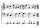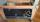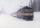# I think

I think a number. When I multiply it by five, and after that I subtract 477, I get the same number as if I multiplied it twice. What number do I think?

Result

x =  159

#### Solution:

5x - 477 = x*2

3x = 477

x = 159

Calculated by our simple equation calculator.

Leave us a comment of example and its solution (i.e. if it is still somewhat unclear...):

Showing 0 comments:Be the first to comment!#### To solve this example are needed these knowledge from mathematics:

Do you have a linear equation or system of equations and looking for its solution? Or do you have quadratic equation?

## Next similar examples:

1. Number unknownAdela thought the two-digit number, she added it to its ten times and got 407. What number does she think?
2. Written numberPlace+values x ten thousands =30 thousands
3. Unknown number xyzFind the number that its triple is 24. Solve by equation.
4. Number of songsWrite an expression for the number of songs they need for this show. Evan and Peter have a radio show that has 2 parts. They need 4 fewer than 11 songs in the first part. In the second part, they need 5 fewer than 3 times the number of songs in the first p
5. What is missing (1000)What number is to add to get 1000?
6. Seven timesWhich number seven times is just as higher as 27, how much is smaller than 29?
7. Roman numerals +Add up the number writtens in Roman numerals. Write the results as a decimal number.
8. Roman numeralsWrite numbers written in Roman numerals as decimal.
9. SummandOne of the summands is 145. The second is 10 more. Determine the sum of the summands.
10. Forest nurseryIn the forest nursery after winter, they found that 1/10 stems died out of them. For them, they land 193 new spruces. How many spruces are in the forest nursery?
11. Jan and DanJan and Dan had the same money. Jan bought 5 workbooks and left him 15 CZK. Dan 6 and left him nothing. How much money have in total?
12. HotelThe rooms in the mountain hotel are double and triple. Double rooms are 25 and triple are 17 more. How many rooms are there in this hotel?
13. If-then equationIf 5x - 17 = -x + 7, then x =
14. Eq1Solve equation: 4(a-3)=3(2a-5)
15. Temperature 2Sunday's high temperature was 3 degrees higher than Saturday's. On Monday, the temperature fell 5 degrees, then rose 7 degrees on Tuesday and 4 more on Wednesday. Then it fell 17 degrees to a record low of 31 on Thursday. What was the temperature on Saturd
16. TrainThe train passes part of the line for 95 minutes at speed 75 km/h. What speed would have to go in order to shorten the driving time of 20 minutes?
17. BenchesThe park has 64 benches. Occupied are by 18 more than empty. How many benches are occupied and empty ?